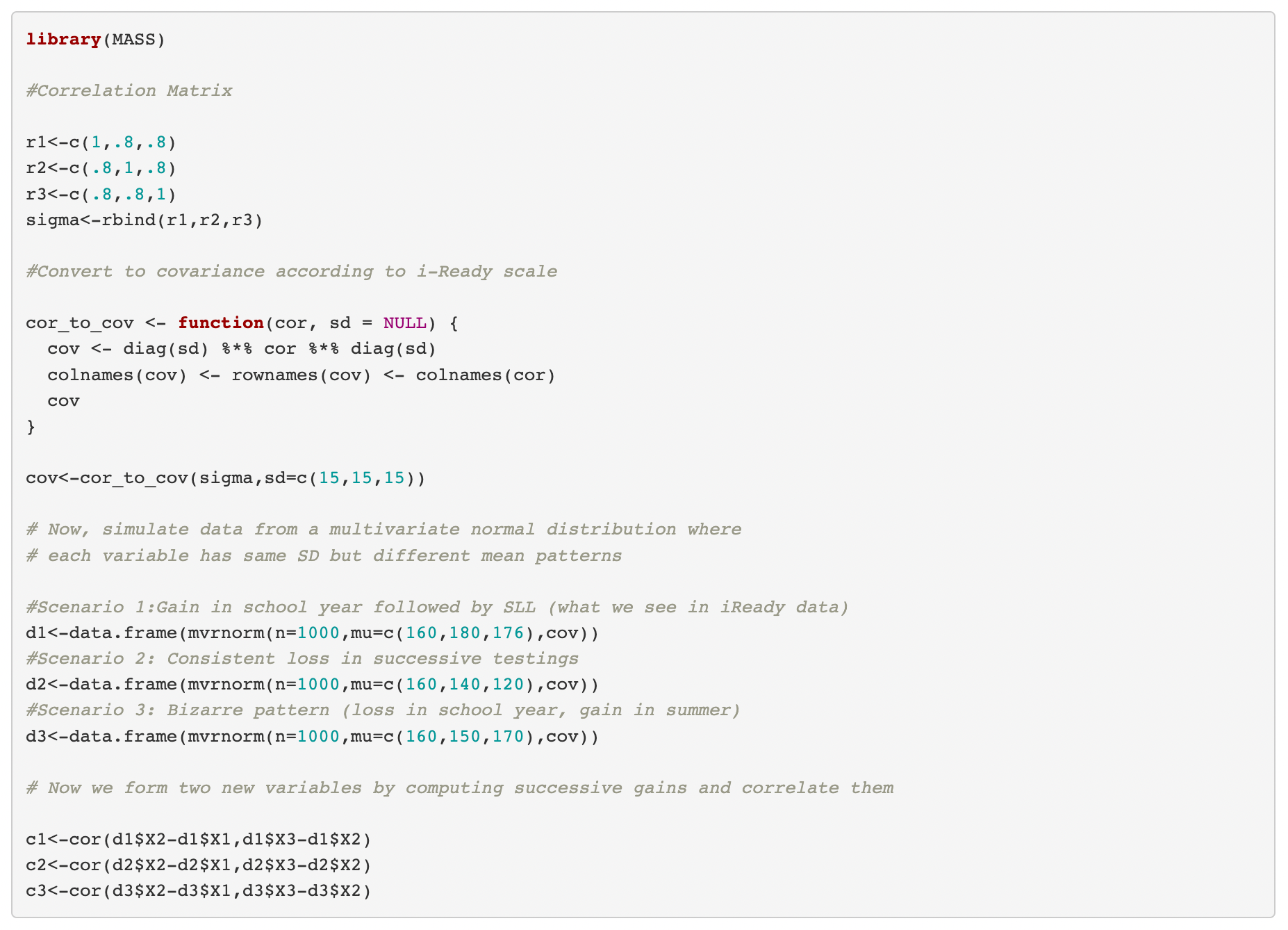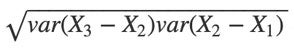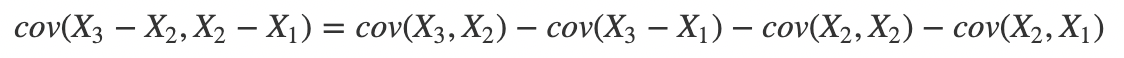Published: March 10, 2022

## School Year Gains and Summer Losses?

In an article for Phi Delta Kappan entitled “Rethinking summer slide: the more you gain, the more you lose” Kuhfeld (2019) uses NWEA MAP test data to show that students with the largest test score gain from fall to spring of an academic school year are those likely to have the largest score declines from spring to the fall of a subsequent school year.

Kuhfeld writes:

“These analyses showed a somewhat surprising result: The strongest predictor of whether a student would experience summer gains or losses was the size of gain the student had made during the previous academic year. That is to say, the more students learned during the school year, the more likely they were to lose ground during summer break. Knowing how much a student gained in the prior year alone explained between 22 and 39% of the variation in summer learning patterns (depending on the grade/subject).”

Kuhfeld concludes the Kappan piece with an unusual policy recommendation:

“According to my own recent studies, an under-examined explanation for summer loss is that spring-to-fall test-score declines tend to be most pronounced for students who had the largest gains from fall to spring. Knowing this, schools that track students’ fall-to-spring learning should be able to identify those students who made above-average gains in the current school year and may be at highest risk for losing ground during the summer.”

In what follows I will use a straightforward simulation to illustrate why such a result is not surprising, but another example of a famous statistical artifact: regression to the mean. Once this is understood, it becomes clear why it would be a rather big mistake for schools to follow the recommendation to identify students showing above average gains during the school year as at high risk for “losing ground” during the summer, just as it would even more ill-advised to ignore students with below average gains because it is assumed that these are the students most likely to “gain ground” during the summer.

## A Simulation

To show this, I will simulate data according to a scenario of “summer learning loss” with population level parameters for MAP test scores that approximate those reported in Kuhfeld, Condron & Downey (2021).

Consider hypothetical students who were in grade 1 as of the fall of 2018. These students tested on three occasions: fall 2018 and spring 2019 of the grade 1 school year, and fall 2019 near the start of their grade 2 school year. The mean scores observed on these occasions for the full population of students are 160, 180 and 176. This indicates that on average, students appear to show growth of 20 MAP scale score units during the school year, but show a decline of 4 units over the summer that follows. Let the standard deviation (SD) on each test occasion be 15 and let the three test scores have the same pairwise correlations of r = .80.

For those interested, the details of the simulation, which also include two other growth scenarios, should be fairly easy to follow in the R code below.I am simulating student scores on each test occasion such that individual differences from the mean for any given student are all a function of chance. By design, there is nothing systematic about individual differences on each test occasion. Given this, if we see the same result described by Kuhfeld–a negative correlation between gain scores–then we know it shouldn’t be given a substantive interpretation.

The figure below, courtesy of my colleague Ben Shear, is illustrative of data simulated under the first of three scenarios.Each colored line in the plot depicts a test score pattern for 10 simulated students. The black line shows the average pattern across a full population of students taking a test three times, twice during a school year, and then once again in the fall of the next school year. The observation that the mean for the fall test is lower than that of the spring is typically take as evidence that students experience “summer slide” or “summer learning loss.”

## Surprising Results?

Here is what we see when we plot the gains from fall 2018 to spring 2019 (x-axis) against the gains from spring 2019 to fall 2019 (y-xis) when the means of the three tests are 160, 180 and 176.Notice that r = -.5.

As it turns out, the mean pattern across test occasions doesn’t matter.

Here is what we get when the means of the three tests are 160, 140 and 120. (The mean score goes down on each occasion)And here is what we get when the means of the three tests are 160, 150 and 170. (The mean score declines during the school year, but increases dramatically after summer.)In fact, given the setup of this simulation with three variables having the same common intercorrelations and SD, a correlation in gain scores of -.5 can be deduced analytically. Credit goes to Andrew Ho for pointing this out. The solution is presented as a technical addendum at the end of this note.

The moral of the story? When you have three variables x, y and z that are positively correlated, and then compute two difference scores that share a common source but in an opposite direction (e.g., diff1 = y-x and diff2 = z-y) we should not be surprised to find that the difference scores (e.g., the “gain scores” diff1 and diff2) are negatively correlated. To ascribe something substantive to this fact (e.g., students who learn more during the school year are most at risk of losing ground in the summer) is to ignore the statistical phenomenon of regression to the mean. This is what Freedman, Pisani & Purves (2007), in their textbook Statistics, refer to as the “regression fallacy.”

## Wait. Where are we seeing regression to the mean in all of this?

Consider the way I simulated a vector of three test scores for each person. Each score is subject to a systematic component (from the constraint that it is one of three scores that have a common intercorrelation) and also a random component (a consequence of drawing at random from the multivariate normal distribution function).

More specifically, from classical test theory we can stipulate the linear model X = T + E. Under this model, when a person has an observed score X that is above or below average for a population of test-takers, this deviation from average can either be because a person is actually above or below average (high or low T), or because the person experienced some good or bad luck (high or low E).

We can’t disentangle T and E for any individual. But what we can plausibly assume is that when a person is observed to score high on X on any single testing occasion, it is more likely that E was positive as opposed to negative. This is what is meant by experiencing “good luck.” So when simulating three scores, if one score is well above average, regression to the mean tells us to expect that the other two scores will also be above average, but not as much (depending upon the magnitudes of the intercorrelations among the three scores).

Therefore, if a student scores above average in the spring, we can predict the student will have a school year gain that is also above average (and vice-versa). Sure enough, in my simulation the correlation between spring score and school year gain is about .3. But a big explanation for these above average gains is measurement error. And if a student experienced good luck in the spring, our best guess is that the student will not experience the same degree of good luck in the fall (and again vice versa for a student who experienced bad luck in the spring). So we can predict a negative correlation between spring scores and spring to fall score differences. And indeed we do. This correlation was -.26 in my simulation. Note the symmetry: the spring score is positively correlated with the first difference, and negatively correlated with the second.

To reiterate the bottom line: a negative correlation between two difference scores that share a common source is a predictable consequence of regression to the mean. As such, when it is observed empirically it should not be described as “an under-examined explanation for summer loss.”

In collaborations with a number of colleagues Kuhfeld has done and continues to produce important scholarship on the topic of modeling and interpreting seasonal patterns and trends in student growth. See Kuhfeld, Gershoff & Paschall (2018); Kuhfeld, Soland, Tarasawa, Johnson, Ryzek, & Liu (2020); and Kuhfeld, Condron, & Downey, (2021). But on this one specific interpretation and recommendation she is missing the mark.

## So what should schools convey to parents about their child’s growth?

Telling parents that a child showed growth of “X scale score units” over the course of the school year is unlikely to be helpful unless the unit of the scale has been made meaningful to parents. This has been a longstanding focus of my own research–see Briggs (2019)–and I’m presently working with my graduate students on an approach I plan to share in the near future. In the absence of internally meaningful scale score units, I would argue that the best possible approach is one in which parents are given concrete examples of differences in the kinds of test questions the child was (and was not) able to answer correctly at time point 2 relative to time point 1, time point 3 relative to time point 2, etc. If questions that a child was solving correctly in the spring are being missed in the subsequent fall (and taken to be evidence of “summer learning loss”), it would be important to know more about the cognitive complexity of the questions. For example, are these questions that mostly emphasize recall or questions that require the application of skills and concepts?

It is also important to be up front with parents about the uncertainty in what can be inferred about the location of an individual student on a test score scale at any one point in time, and about the growth of a student across multiple points in time. For example, the grade 1 MAP math scale ranges from about 100 to 200. Let’s assume that the marginal reliability coefficient for a MAP score on any given occasion is .95. This means we can predict that the average standard error of measurement associated with a score location within this range for children who take a test in the spring will be about 3.4 points, and if measurement error is normally distributed, then a 95% confidence interval around a student’s score will encompass about 7 points in either direction.

The uncertainty associated with measurement error is even greater when it comes to gain scores. For grade 1 students, let’s say the mean gain from fall to spring of a school year is 20 points (as in the simulation). But the standard error of measurement associated with this gain score will be about 5 points. For any individual student with an observed gain equal to the mean of 20, the “true gain” could be as low as 10 and as high as 30. To put this in perspective, a 10 point gain would quite likely place the student below the bottom quartile of the observed gain score distribution, while a 30 point gain would likely put the student in the top quartile!

In other words, given what we can infer about the magnitude of measurement error in testing, it would not be surprising to frequently observe what appears to be a negative gain. This can be the case even when the true gain–the quantity we would observe if it were possible to repeatedly administer different fall and spring tests and compute the average of the resulting distribution of differences–is positive. So teachers and administrators at a school would want to tread carefully before overreacting to an apparent gain or loss across two test occasions. There is surely some signal in this information–especially when it is being aggregated to the level of a classroom or school. But there is also a considerable amount of noise.

Finally, there is a last source of uncertainty that should be acknowledged, and that is the limited reach of any test instrument–no matter how carefully developed–to measure the breadth and depth of what children are learning in and out of school settings. This is a source of uncertainty that can’t be readily quantified with a mathematical model, but to a great extent it is the more important source to recognize. Even with the availability of a vertical scale and computer adaptive item administration, it is a fairly audacious claim to observe that a student’s score on a fall testing is lower than the score observed on a prior spring testing and refer to this as evidence of “learning loss.” The observation may well be a greater indictment of what it is that the test is (and is not) measuring than it is of the academic and intellectual progress of any given child.

## Acknowledgments

I thank Mimi Engel, Andrew Ho, Kristen Huff, Ben Shear, and Sandy Student for their comments and contributions to this note.

## References

Briggs, D. C. (2019). Interpreting and visualizing the unit of measurement in the Rasch Model. Measurement, 46 (2019) 961–971. https://doi.org/10.1016/j.measurement.2019.07.035

Freedman, D., Pisani, R., & Purves, R. (2007). Statistics, 4th Edition. New York: W. W. Norton & Company.

Kuhfeld, M., Gershoff, E. & Paschall, K. (2018). The development of racial/ethnic and socioeconomic achievement gaps during the school years. Journal of Applied Developmental Psychology, 57, 62-73. https://doi.org/10.1016/j.appdev.2018.07.001

Kuhfeld, M. (2019). Surprising new evidence on summer learning loss. Phi Delta Kappan, 101(1), 25-29.

Kuhfeld, M., Soland, J., Tarasawa, B., Johnson, A., Ruzek, E., & Liu, J. (2020). Projecting the potential impact of COVID-19 school closures on academic achievement. Educational Researcher, 49(8), 549-565.

Kuhfeld, M., Condron, D. J., & Downey, D. B. (2021). When Does Inequality Grow? A Seasonal Analysis of Racial/Ethnic Disparities in Learning From Kindergarten Through Eighth Grade. Educational Researcher, 50(4), 225–238. https://doi.org/10.3102/0013189X20977854

## Technical Appendix

### Analytic derivation of the negative correlation of -.5

Let X1X2, and X3 be the test scores on three successive occasions and represent them as three random variables with a multivariate normal distribution. For simplicity, I assumed they have the same variance and covariance.In the present setup we would typically assume that x and y represent some finite positive real value (i.e., test score variance is greater than 0 and tests have positive covariance across occasions). Note that in the special case of three variables that are multivariate standard normal, x = 1 and y = r, where r is just a correlation coefficient.

To find the variance in gain scores from occasion 1 to 2 and 2 to 3 we needNow by definition, the correlation between the two gain scores isThe denominatorsimplifies to 2x − 2y

The numerator can be expanded and written aswhich simplified to yx

Thus### Latent growth curves

In an early version of this note that I shared with Megan Kuhfeld, I claimed (erroneously as it turns out) that any time we have three variables with positive intercorrelations, the correlation between X− X1 and X− X2  will be negative. She proved me wrong by generating data from a latent growth curve model in which each simulated student comes from a population with a positive linear trajectory across four occasions. It can be shown in this setup that simulated test scores will be positively correlated across occasion, and that the gain scores are positively correlated as well. I thought this was quite clever.

However, as I pointed out in my response: (1) the resulting correlations that are generated in such an exercise have little resemblance to the actual patterns we observe for MAP scores across temporal occasions, and (2) the approach Kuhfeld took in her 2019 article in question, because it relies on a piecewise growth model (all growth parameters are based on just two occasions), is unable to disentangle an underlying growth trajectory from the effect of regression to the mean.

How to cite: